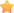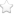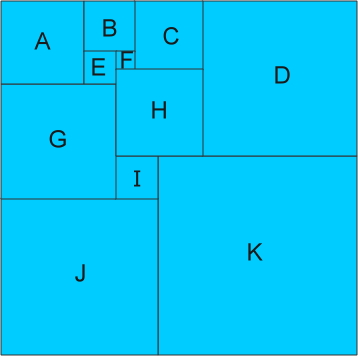×A=41，B=25，C=34，D=77，E=16，F=9，
G=57，H=43，I=21，J=78，K=99

A=B+E；B=E+F；C=B+F；D=C+H；G=A+E；H=C+F；J=G+I；K=I+J

A=2E+F；B=E+F；C=E+2F；D=2E+5F；G=3E+F；H=E+3F；J=3E+F+I；K=3E+F+2I

J+K=177 → 6E+2F+3I=177 → 12E+4F+6I=354
K+D=176 → 5E+6F+2I=176 → 15E+18F+6I=528

F可能是3, 6, 9，所以(E, F)只有(44, 3), (30, 6), (16, 9)這三種可能

J+K=177 → 6E+2F+3I=177 → 6x16+2x9+3I=177 → I=21

E=16, F=9, I=21，其他正方形的邊長就都可以用這三個值算出來啦！

（你有更好的解法嗎？歡迎到粉絲團遊戲學校去跟大家分享喔！）